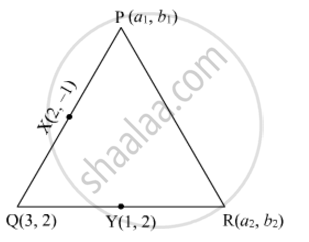# Find the area of the triangle PQR with Q(3,2) and the mid-points of the sides through Q being (2,−1) and (1,2). - Mathematics

Find the area of the triangle PQR with Q(3,2) and the mid-points of the sides through Q being (2,−1) and (1,2).

#### Solution

Let the coordinates of the vertices P and R of ∆PQR be (a1, b1) and (a2, b2), respectively.Suppose X(2, 1) is the midpoint of PQ.

Then,

(2,-1)=((a_1+3)/2, (b_1+2)/2)

=>(a_1+3)/2=2

a1=1 and b1=4

Therefore, the coordinates of P are (1, −4).

Again, suppose Y(1, 2) is the midpoint of QR.

Now,

(1,2)=((a_2+3)/2,(b_2+2)/2)

=>(a_2+3)/2=1

a2=1 and b2=2

Therefore, the coordinates of R are (−1, 2).

Thus, the vertices of ∆PQR are P(1,−4), Q(3, 2) and R(−1, 2).

Now,

Area of PQR =1/2×[1(22)+3(2+4)1(42)]

=1/2(18+6)

=1/2(24)

=12

Thus, the area of ∆PQR is 12 square units.

Concept: Area of a Triangle
Is there an error in this question or solution?
Chapter 6: Co-Ordinate Geometry - Exercise 6.5 [Page 54]

#### APPEARS IN

RD Sharma Class 10 Maths
Chapter 6 Co-Ordinate Geometry
Exercise 6.5 | Q 8 | Page 54
Share